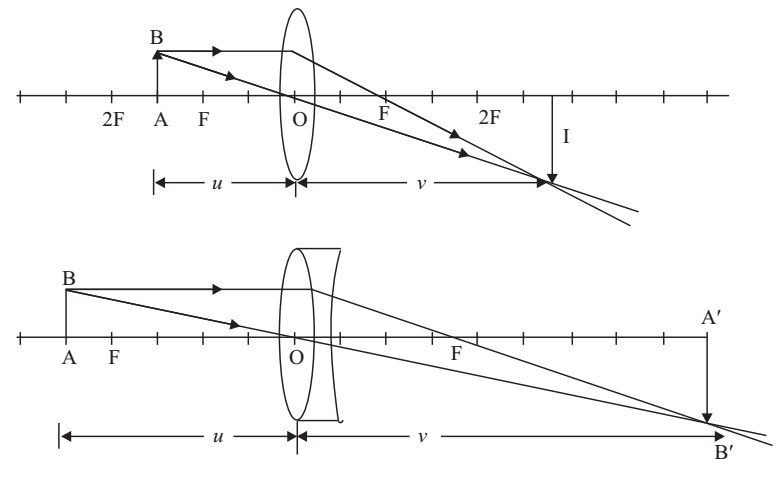# Focal Length of Concave Lens Experiment

Experiment: Determine the focal length of a concave lens by combining it with a suitable convex lens.

If two lenses of fecal lengths f1 and f2 are kept in contact, the focal length F of the combination is given by

1/F = 1/f1 + 1/f2

As per new cartesian sign convention, focal length of a concave lens is negative and the focal length of a convex lens is positive. Hence if the first lens is convex and the second is concave then the focal length of the combination of these two lenses is given by

1/F = 1/f1 - 1/f2

F = f1f2/(f2-f1)

If f2 is more than f1, the focal length of the combination will be positive and hence it will behave as a converging (convex) lens.

Using two pin method, determine the focal length of the convex lens f1 and the focal length of the combination F. The focal length of the concave lens may be determine using the formula

f2 = f1F/(f1-F)

Material Required

Optical bench with three uprights, knitting needle, two pins, lens holder, concave lens, convex lens, cellotape, spirit level, half metre rod

Lenses should be so chosen so that the focal length of the combination should not be greater than 20 cm, e.g. 10 cm and – 20 cm.

### How To Set Up

1. Level the optical bench with the help of spirit level and leveling screws.

2. Check that the convex lens in contact with the given concave lens forms an enlarged image of a nearby object. If it makes a diminished image, change the convex lens with another one of shorter focal length.3. Fix the convex lens in the middle of the optical bench and place one pin on either side of it. Adjust the centre of the lens and the tips of the pins in the same horizontal line.

### How To Perform Experiment

1. Determine the rough focal length of the convex lens with the help of metre scale, by. forming the image of a distant object on the wall.

2. Fix the lens in the lens holder in the middle upright and place the object-pin AB at a distance greater than rough focal length on one side of the lens.

3. Move the image pin and remove parallax between its tip of and the image of AB through the lens i.e. A1 B1.

4. Note the index corrected values of u and v.

5. Repeat the experiment for four or five different values of u.

6. Put the concave lens in contact with the convex lens. Fix them together with the help of cellotape at the rim.

7. Repeat the procedure for determining the focal length of a convex lens.